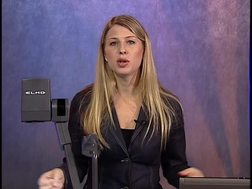PLAY PREVIEW

### Segments in this Video

#### Outline: Independent T-Tests: Lecture 8(01:38)

FREE PREVIEW

In this lecture, Prof. Naomi Lowe will explain the types of T-tests; hypothesis, distribution of mean differences, and calculations of independent T-tests; effect size; and issues with T.

#### Types of T-Tests(02:21)

Types include: one-sample, dependent (paired) T, and independent (two sample) T.

#### Independent T: Hypothesis Testing(11:09)

Compare two samples using sample information from both populations; scores are independent from each other. The types of variables for these T-tests are nominal two-level and continuous. See examples using S.I. and exam scores, and two identical populations.

#### Independent T: Distribution of Mean Differences(07:57)

Use information from previous calculations. If the null is true, both populations have equal means; the T-distribution will center around zero. See an example involving students reading their names in a story.

#### Independent T: Spread of SD of Mean Differences(10:56)

Calculating standard error takes several steps—comparison distribution, population variances, pooled variances, pooled distribution of means, variance distribution of means, and standard distribution of the differences of means (S.E.). See examples of each step.

#### Independent T: Calculations Using Pooled Standard Error(07:10)

Calculate the T-score and examine the cut-off on the T-distribution. Plot calculations on the diagram and determine whether to accept or reject the null.

#### Independent T: Assumptions(02:12)

T-test assumptions are that the populations are normally distributed and the variance is equal—homogeneity of variance (HOV).

#### Effect Size(01:31)

Effect size for the sampling of independent T-testing requires number substitution; it measures the size of the difference between groups. See the formula for estimating effect size.

#### Issues With T(02:36)

Cut-offs in the T-table assume one is performing a single T-test. Running multiple tests with the same data can result in a false rejection of the null.

For additional digital leasing and purchase options contact a media consultant at 800-257-5126
(press option 3) or sales@films.com.

# Independent T-Tests: Lecture 8

Part of the Series : Statistics With Naomi Lowe
 DVD (Chaptered) Price: \$129.95 DVD + 3-Year Streaming Price: \$194.93 3-Year Streaming Price: \$129.95

Share

### Description

In this video lecture, Professor Naomi Lowe explains Independent T-Tests.

Length: 48 minutes

Item#: BVL150108

ISBN: 978-1-64347-560-8Closed Captioned